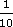Squares, roots and powers

This free course is available to start right now. Review the full course description and key learning outcomes and create an account and enrol if you want a free statement of participation.

Free course

2.2.1 Try some yourself

Activity 16

Find the following powers by hand, as estimates for calculator work.

• (a) 107

• (b) 108

• (c) 34

• (d) (2)2

• (e) (2)3

• (a) 107 = 10 000 000

• (b) 108 = 100 000 000

• (c) 34 = 3 × 3 × 3 × 3 = 27 × 3 = 81

• (d) (2)2 = 2 × 2 = 4

• (e) (2)3 = 4 × 2 = 8

Activity 17

• (a) 117

• (b) 10.88

• (c) 3.144

• (d) (2.2)2

• (e) (2.01)3

• (a) 117 = 19 487 17119 500 000 (3 s.f.)

• (b) 10.88 = 185 093 021185 000 000 (3 s.f.)

• (c) 3.144 = 97.2117121697.2 (3 s.f.)

• (d) (2.2)2 = 4.84

• (e) (2.01)3 = 8.1206018.12 (3 s.f.)

Activity 18

• (a) How many zeros are there in 1012 when written out in full? Work out a rule for finding the number of zeros in any positive whole number power of 10.

• (b) Your answers to parts (d) and (e) of Question 1 may have suggested a rule for powers of negative numbers, depending on whether the power is odd or even. What is the rule?

• (a) There are 12 zeros in 1012. The number of zeros for a positive power of 10 is the same as the power.

• (b) An odd power of a negative number is negative. An even power of a negative number is positive.

Activity 19

Hamsters of a particular kind, left to breed in a suitable environment, double their population every month. If a friend of yours, who is thinking of breeding these hamsters, started with one pair (male and female), what population might she expect in a year's time (assuming she keeps them all)?

After 0 months (i.e. at the start) there are 2 hamsters = 2

After 1 month there are 2 × 2 hamsters, i.e. 22 = 4

After 2 months there are 2 × 2 × 2 hamsters, i.e. 23 = 8

So after 12 months there are 213 = 8192 hamsters.

Activity 20

A ploughed field has been left abandoned. It has one weed in the middle. The weed is an annual plant and its flower produces about 1000 seeds. If a tenth of these seeds germinate to produce one flower each in a year's time, estimate the possible number of dandelion flowers in the field if it is abandoned for five years. (You can assume that no other factors affect the growth of the weeds during this time).of 1000 = 100

So 100 seeds per flower germinate each year.

At 0 years there is 1 weed.

After 1 year there are 1 × 100 = 100 weeds (which can be written as 1001).

After 2 years there are

100 × 100 = 1002 = 10 000 weeds.

So after 5 years an estimate is

1005 = 10 000 000 000 weeds (or 10 billion).

Activity 21

Cells in a laboratory culture divide into two every day, given sufficient nutrient. If there were five cells on day one, how many would there be on day ten?

On day 1 there are 5 cells

On day 2 there are 5 × 2 = 10 cells

On day 3 there are 5 × 2 × 2 = 5 × 22 = 20 cells

On day 4 there are 5 × 2 × 2 × 2 = 5 × 23 = 40 cells.

So on day 10 there are 5 × 29 = 5 × 512 = 2560 cells.

MU120_4M4